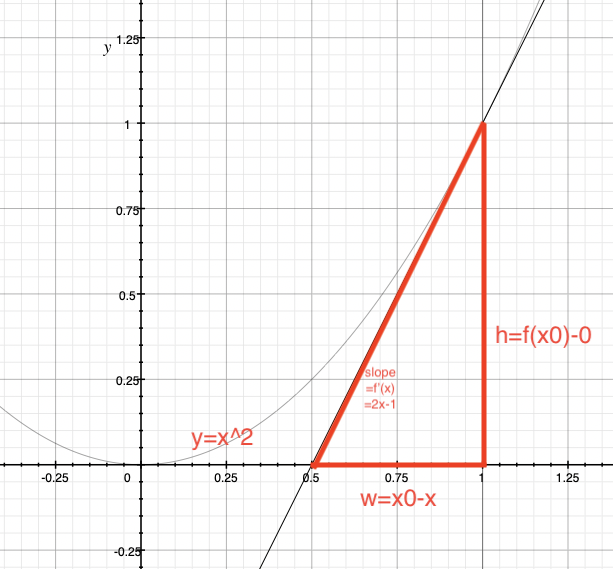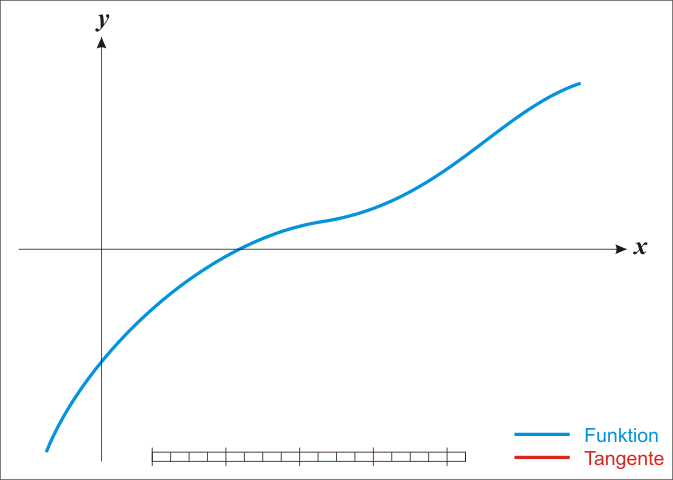# Newton Iteration Method

2020-08-31f(x) / (x0 - x) = f'(x), 即 x0 - x = f(x) / f'(x).package main

import (
"fmt"
"math"
)

const PRECISION float64 = 0.0000000001
const START_SPECULATE = 1.0

func Sqrt(x float64) (float64, int) {
times := 0
z := START_SPECULATE
for !(math.Abs(x-z*z) <= PRECISION) {
z += -1 * (z*z - x) / (2 * z)
times += 1
}
return z, times
}

func main() {
var z float64
var t int

z, t = Sqrt(2)
fmt.Printf("Sqrt(2): %.9f\ttimes: %d\n", z, t)

z, t = Sqrt(9)
fmt.Printf("Sqrt(9): %.9f\ttimes: %d\n", z, t)
}



output:

Sqrt(2): 1.414213562	times: 4
Sqrt(9): 3.000000000	times: 6


START_SPECULATE 是我们迭代的起始坐标, 理论上来说可以随便取.

## 参考

https://zh.wikipedia.org/zh-hans/%E7%89%9B%E9%A1%BF%E6%B3%95